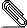[racket-dev] FFI question; using _or-null around output ptr gives binding error.

Here's an ffi object I'd like to construct:

(define do-fit-int
(get-ffi-obj "do_fit" libfit
(_fun (func      : (_fun _int _pointer -> _double))
(val-num   : _int = (length x-values))
(x-values  : (_list i _double*))
(y-values  : (_list i _double*))
(z-values  : (_list i _double*))
(errors    : (_list i _double*))
(param-num : _int = (length params))
(params    : (_list i _double*))
-> (_or-null (_list o _double* param-num)))))

My addition is the (_or-null ...) that's wrapped around the output list, and
this signals the error:

expand: unbound identifier in module in: param-num

It looks like I can work around this error by doing the work explicitly:

(define do-fit-int
(get-ffi-obj "do_fit" libfit
(_fun (func      : (_fun _int _pointer -> _double))
(val-num   : _int = (length x-values))
(x-values  : (_list i _double*))
(y-values  : (_list i _double*))
(z-values  : (_list i _double*))
(errors    : (_list i _double*))
(param-num : _int = (length params))
(params    : (_list i _double*))
-> (result : _pointer)
-> (and result
(cblock->list result _double* param-num)))))

...which compiles, though I can't say anything yet about whether it runs
correctly (principally because I haven't yet found a set of inputs on which the
fit library doesn't choke).

My question: should or-null handle this?

Johnsmime.p7s
Description: S/MIME cryptographic signature

_________________________________________________